Notebook

During the "Quant Crash" of Aug 7-9, 2007, numerous fundamental factors, like value factors, performed extraordinarly poorly, but a simple short-term mean-reversion strategy held up better. I'll try to demonstrate this in this notebook.

In :
# Import necessary Pipeline modules
import pandas as pd
import numpy as np
from matplotlib import pyplot as plt
from quantopian.pipeline import Pipeline
from quantopian.research import run_pipeline
from quantopian.pipeline.factors import AverageDollarVolume
from quantopian.pipeline.data import morningstar as mstar
from quantopian.pipeline.factors import CustomFactor
from quantopian.pipeline.data.builtin import USEquityPricing
from quantopian.pipeline.filters import Q500US,Q1500US


Get data on returns during various windows around the Quant Crash, as well as a snapshot of cash flow-to-price ratios.

In :
# Returns on Aug 7,8,9
class CrashReturn(CustomFactor):
inputs = [USEquityPricing.close]
window_length=5

def compute(self, today, assets, out, close):
out[:] = np.log(close[-2]) - np.log(close)

# Returns on Aug 9
class LastDayCrashReturn(CustomFactor):
inputs = [USEquityPricing.close]
window_length=5

def compute(self, today, assets, out, close):
out[:] = np.log(close[-2]) - np.log(close[-3])

# Returns on Aug 10
class DayAfterCrashReturn(CustomFactor):
inputs = [USEquityPricing.close]
window_length=2

def compute(self, today, assets, out, close):
out[:] = np.log(close[-1]) - np.log(close)

# Returns on five days before Aug 7, but skipping Aug 6
class FiveDayReturn(CustomFactor):
inputs = [USEquityPricing.close]
window_length=10

def compute(self, today, assets, out, close):
out[:] = np.log(close[-6]) - np.log(close)

# Returns on five days before Aug 7, not skipping Aug 6
class FiveDayReturnNoSkip(CustomFactor):
inputs = [USEquityPricing.close]
window_length=10

def compute(self, today, assets, out, close):
out[:] = np.log(close[-5]) - np.log(close)

# Returns on five days before Aug 9, but skipping Aug 8
class FiveDayReturnBeforeLastDay(CustomFactor):
inputs = [USEquityPricing.close]
window_length=10

def compute(self, today, assets, out, close):
out[:] = np.log(close[-4]) - np.log(close[-8])

# Returns on five days before Aug 9, not skipping Aug 8
class FiveDayReturnBeforeLastDayNoSkip(CustomFactor):
inputs = [USEquityPricing.close]
window_length=10

def compute(self, today, assets, out, close):
out[:] = np.log(close[-3]) - np.log(close[-8])

class CF_To_Price(CustomFactor):
inputs=[mstar.valuation_ratios.cf_yield]
window_length = 5

def compute(self,today,assets,out,cf_yield):
out[:]=cf_yield

pipe = Pipeline(
columns={
'CrashReturn': CrashReturn(),
'LastDayCrashReturn': LastDayCrashReturn(),
'DayAfterCrashReturn': DayAfterCrashReturn(),
'FiveDayReturn': FiveDayReturn(),
'FiveDayReturnNoSkip': FiveDayReturnNoSkip(),
'FiveDayReturnBeforeLastDay': FiveDayReturnBeforeLastDay(),
'FiveDayReturnBeforeLastDayNoSkip': FiveDayReturnBeforeLastDayNoSkip(),
'CF_To_Price': CF_To_Price(),
'sector': mstar.asset_classification.morningstar_sector_code.latest
},
#screen= (Q1500US() & ~Q500US())
screen= (Q1500US())
)
start_date = '2007-08-13'
end_date = '2007-08-13'

data = run_pipeline(pipe, start_date, end_date)
data.index=data.index.droplevel(0)

Out:
CF_To_Price CrashReturn DayAfterCrashReturn FiveDayReturn FiveDayReturnBeforeLastDay FiveDayReturnBeforeLastDayNoSkip FiveDayReturnNoSkip LastDayCrashReturn sector
Equity(2 [ARNC]) 0.1182 -0.005058 -0.022505 -0.047991 -0.038095 -0.021014 -0.062736 -0.046776 101
Equity(24 [AAPL]) 0.0414 -0.064070 -0.014297 -0.071367 0.000000 -0.008027 -0.045972 -0.055229 311
Equity(62 [ABT]) 0.0658 0.041357 -0.000362 0.004910 0.062259 0.096093 0.043535 -0.016503 206
Equity(67 [ADSK]) 0.0633 0.051016 -0.010831 -0.078788 -0.018247 0.008660 -0.055361 -0.010034 311
Equity(76 [TAP]) 0.0819 -0.114137 0.006674 0.020491 0.008276 -0.036446 0.035153 -0.057835 205

Demean cash flow-to-price by sector

In :
data['CF_To_Price']=data.groupby('sector')['CF_To_Price'].transform(lambda x: x-x.mean())


Conpute deciles for returns and CF/P

In :
data['CF_decile']=pd.qcut(data['CF_To_Price'],10,labels=False)+1
data['CR_decile']=pd.qcut(data['CrashReturn'],10,labels=False)+1
data['FD_decile']=pd.qcut(data['FiveDayReturn'],10,labels=False)+1
data['NS_decile']=pd.qcut(data['FiveDayReturnNoSkip'],10,labels=False)+1
data['FD1_decile']=pd.qcut(data['FiveDayReturnBeforeLastDay'],10,labels=False)+1
data['NS1_decile']=pd.qcut(data['FiveDayReturnBeforeLastDayNoSkip'],10,labels=False)+1


First, we sort stocks into ten cash flow-to-price deciles. Decile 10 represents the traditional value stocks and decile 1 are the growth stocks. Many have argued that traditional factors became very overcrowded. The chart below shows the average returns, over the three-day Quant Crash period of Aug 7-9, for the stocks in each cash flow-to-price decile. The returns are not only montonic in deciles, but extremely large: over those three days, the top decile underperformed the bottom decile by over 7%.

In :
CF_quint_ret=data.groupby('CF_decile')['CrashReturn'].apply(lambda x: x.mean())
CF_quint_ret.plot(kind='bar')
plt.xlabel('CF/P Decile')
plt.ylabel('Returns on Aug 7-9, 2007');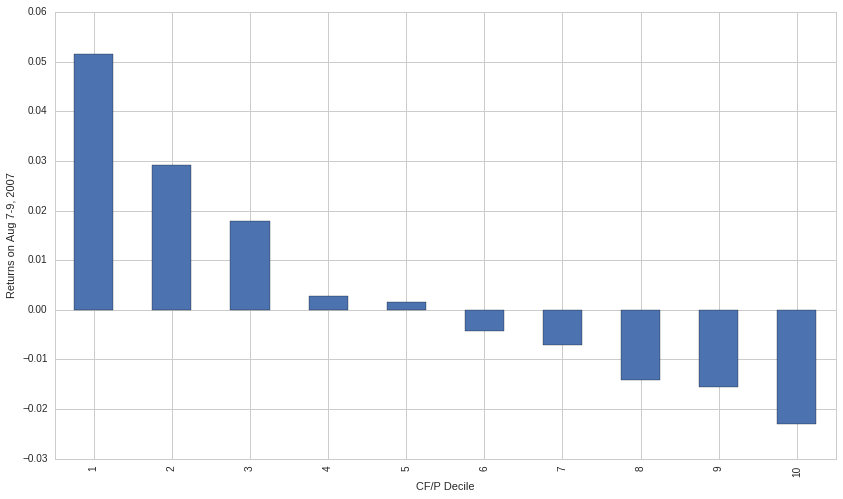Much of those losses reversed the next day. On Aug 10, value outperformed growth by over 4%.

In :
CF_quint_ret=data.groupby('CF_decile')['DayAfterCrashReturn'].apply(lambda x: x.mean())
CF_quint_ret.plot(kind='bar')
plt.xlabel('CF/P Decile')
plt.ylabel('Returns on Aug 10, 2007');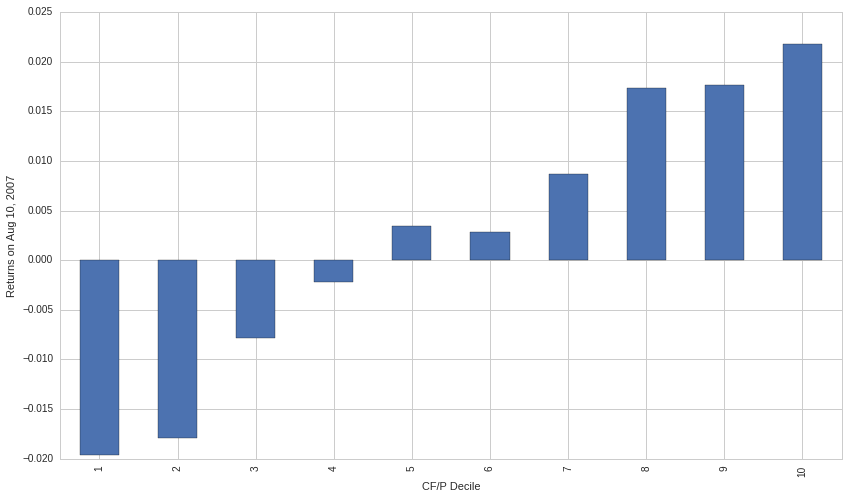Mean reversion held up much better than cash flow-to-price during the Quant Crash, especially among Q500US stocks. Below, we sort stocks into deciles based on the five day returns leading up to the Quant Crash. This was done two ways, following the "Enhancing Mean Reversion Strategies" post. Stocks were sorted using the five-day returns from July 30 to Aug 6, both skipping and not skipping the Aug 6 returns. The chart below shows the returns to those portfolios if the stocks were held over the entire Aug 7-9 period (of course, this is for illustrative purposes only, because the short-term mean-reversion strategy would be adding and unwinding positions over the course of those three days, and here we assume positions remain static). There is not much difference in returns between the loser portfolios and the winner portfolios.

In :
FD_quint_ret=data.groupby('FD_decile')['CrashReturn'].apply(lambda x: x.mean())
NS_quint_ret=data.groupby('NS_decile')['CrashReturn'].apply(lambda x: x.mean())
FD_quint_ret.plot(kind='bar', color='red',  position=0, width=0.25,label='Skip')
NS_quint_ret.plot(kind='bar', color='blue',  position=1, width=0.25,label='No Skip')
plt.xlabel('Decile')
plt.ylabel('Return Over Aug 7-9, 2007')
plt.legend(loc='best');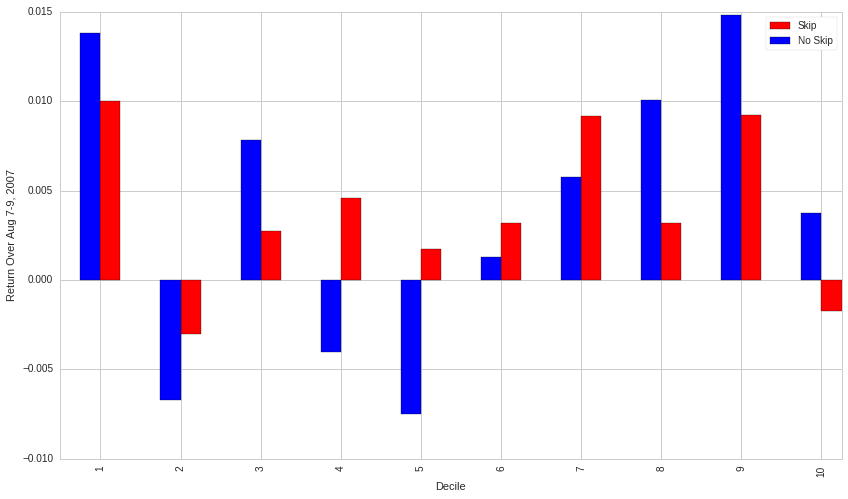The simple mean reversion strategy does not do as well if we sort stocks before the third day of the Quant Crash, August 9, since many of the same stocks that dropped on August 7 and 8 continued to drop on August 9. Note that all decile returns are negative because the overall market dropped by about 3% that day (unlike the prior two days of the Quant Crash, which saw some very large moves in individual stocks but the overall market was relatively flat).

In :
FD1_quint_ret=data.groupby('FD1_decile')['LastDayCrashReturn'].apply(lambda x: x.mean())
NS1_quint_ret=data.groupby('NS1_decile')['LastDayCrashReturn'].apply(lambda x: x.mean())
FD1_quint_ret.plot(kind='bar', color='red',  position=0, width=0.25,label='Skip')
NS1_quint_ret.plot(kind='bar', color='blue',  position=1, width=0.25,label='No Skip')
plt.xlabel('Decile')
plt.ylabel('Return On Aug 10, 2007')
plt.legend(loc='best');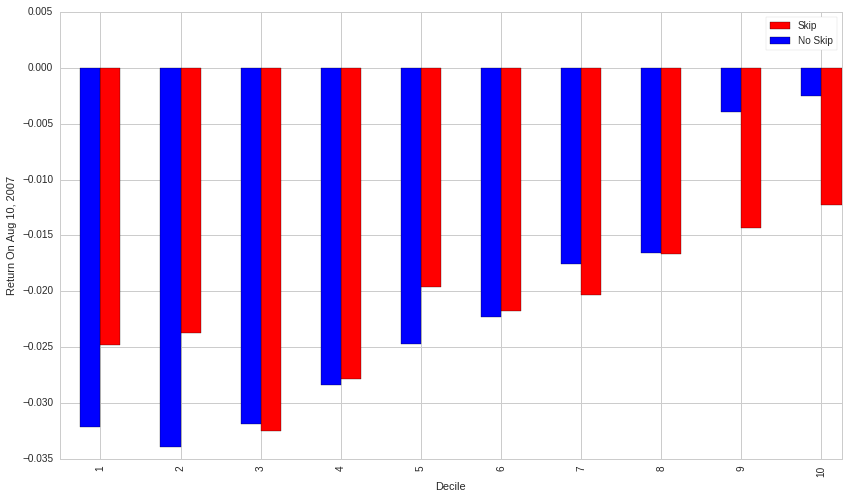CTL was a typical value stock. It's in the landline busines with high cash flow-to-price (decile 10) and low growth. It dropped 10% during the crash, and then recovered, although in this case there was not a big reversal the next day, August 10.

In :
print 'CF/P Decile: ', data.loc[symbols('CTL')].CF_decile

CF/P Decile:  10.0

In :
single_name=get_pricing(symbols('CTL').sid,fields='close_price',start_date='2007-Jun-9',end_date='2007-Aug-31')
single_name.plot(marker='o');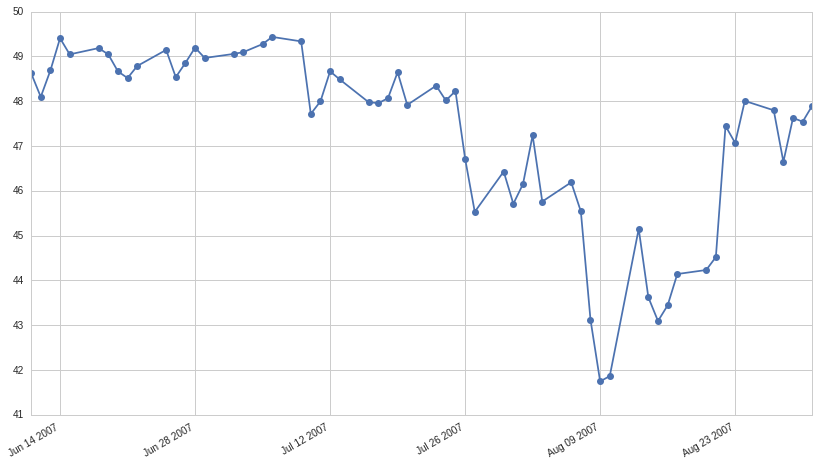Another fun stock to look at is TR (Tootsie Roll). You can't get more low-tech than that, and there were no new product launches, no new flavors of Tootsie Rolls, and in fact no significant news stories that week in August. Yet the stock, which was not that volatile in June and July, jumped over 20% during the crash and gave back almost the entire gain the next day.

In :
single_name=get_pricing(symbols('TR').sid,fields='close_price',start_date='2007-Jun-9',end_date='2007-Aug-31')
single_name.plot(marker='o');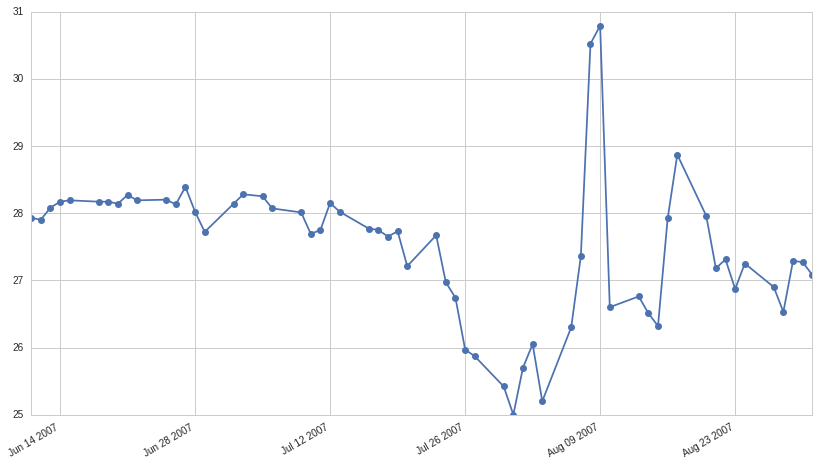In [ ]: